Courses

# Test: Visualising Solid Shapes- 3

## 20 Questions MCQ Test Mathematics (Maths) Class 8 | Test: Visualising Solid Shapes- 3

Description
This mock test of Test: Visualising Solid Shapes- 3 for Class 8 helps you for every Class 8 entrance exam. This contains 20 Multiple Choice Questions for Class 8 Test: Visualising Solid Shapes- 3 (mcq) to study with solutions a complete question bank. The solved questions answers in this Test: Visualising Solid Shapes- 3 quiz give you a good mix of easy questions and tough questions. Class 8 students definitely take this Test: Visualising Solid Shapes- 3 exercise for a better result in the exam. You can find other Test: Visualising Solid Shapes- 3 extra questions, long questions & short questions for Class 8 on EduRev as well by searching above.
QUESTION: 1

Solution:
QUESTION: 2

Solution:
QUESTION: 3

### What is the flat surface of a solid figure called?

Solution:
QUESTION: 4

What is the number of flat surfaces of a cone?

Solution:
QUESTION: 5

Of which shape are the faces of a cuboid?

Solution:
QUESTION: 6

What is the number of corners in a cone?

Solution:
QUESTION: 7

What is the number of corners in a cylinder?

Solution:
QUESTION: 8

What do you call solid figures with line segments as their edges?

Solution:
QUESTION: 9

What is the difference between a rectangle and a cube?

Solution:
QUESTION: 10

How many edges does a cuboid have?

Solution:
QUESTION: 11

Of what shape are the bases of a cylinder?

Solution:
QUESTION: 12

Which of the following statements is false?

Solution:
QUESTION: 13

What does the following figure represent?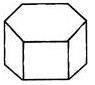Solution:
QUESTION: 14

A rectangular photoframe is an example of which of the following?

Solution:
QUESTION: 15

What is the shape of the given tent?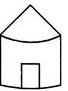Solution:
QUESTION: 16

What is the shape of a sharpened pencil?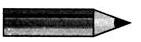Solution:
QUESTION: 17

Which of the following is the side view of a 5 kg weight given?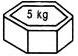Solution:
QUESTION: 18

A solid is of the shape given.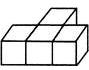Which is its top view?

Solution:
QUESTION: 19

Which of the following objects has the given figure as the front view?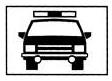Solution:
QUESTION: 20

Observe the following solid.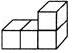The following box has a figure which is a view of the given solid.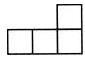Which view of the solid is shown in the box?

Solution: